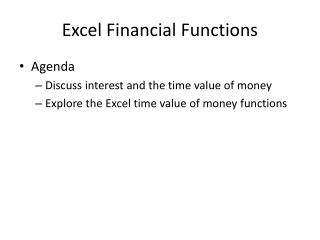DownloadDownload PresentationExcel Financial Functions

# Excel Financial Functions

Download Presentation## Excel Financial Functions

- - - - - - - - - - - - - - - - - - - - - - - - - - - E N D - - - - - - - - - - - - - - - - - - - - - - - - - - -
##### Presentation Transcript

1. Excel Financial Functions • Agenda • Discuss interest and the time value of money • Explore the Excel time value of money functions

2. Working with Financial Functions • Cost of a loan to the borrower is largely based on three factors: • Principal: amount of money being loaned • Interest: amount added to the principal by the lender • Calculated as simple interest or as compound interest • Time required to pay back the loan

3. Using Functions to Manage Personal Finances

4. Excel Functions are Excel Functions To use them, you must understand the TIME VALUE OF MONEY

5. Understanding time value of money Money will increase in value over time if the money is invested and can make more money. If you have \$1,000 today, it will be worth more tomorrow if you invest that \$1,000 and it earns additional money (interest or some other return on that investment). If you have \$1,000 today, it will NOT be worth more tomorrow if you put it in an envelope and hide it in a drawer. Then the time value of money does not apply as an increase. It will most likely decrease in value because of inflation. Of course, you won’t lose the whole \$1,000 either…

6. Introduction to Interest Calculations • When you borrow money you pay interest • When you loan money, you receive interest • When you make a payment • part of the payment is applied to interest • Part of the payment is applied to principal

7. Types of Interest • Simple interest • Interest is paid only on the principal • Many certificates of deposit work this way • Compound interest • Interest is added to the principal each period • Interest is calculated on the principal plus any accrued interest • Compounding can occur on different periods • Annually, quarterly, monthly, daily

8. Difference between simple and compound interest Assume that you have \$1,000 to invest. \$1,000 is the present value (PV) of your money. You can invest it and receive “simple” interest or you can earn “compound” interest. The money that you have at the end of the time you have invested it is called the “future value” (FV) of your money.

9. Future value of money Simple interest is always calculated on the initial \$1,000. 5% interest on \$1,000 is \$50. Always \$50. When interest is paid on not only the principal amount invested, but also on any previous interest earned, this is called compound interest. FV = Principal + (Principal x Interest) = 1000 + (1000 x .05) = 1000 (1 + i) = PV (1 + i)

10. Simple vs. compound interest comparison \$1,000 Invested at 5% return

11. Time Value of Money Functions • We are just solving the same equation for a different variable • RATE determines the interest rate • NPER determines the number of periods • PMT determines the payment • PV determines the present value of a transaction • FV determines the future value of a transaction

12. Future Value Function FV(rate, nper, pmt, [pv], [type])

13. Present Value Function PV(rate, nper, pmt, [fv], [type])

14. Payment function PMT(rate, nper, pv, [fv], [type])

15. The RATE Function • Determines the interest rate PER PERIOD based on • The number of periods • The payment • The present value • The future value • The type

16. The NPER Function • Determines the number of periods based on • The interest rate • The payment • The present value • The future value • The type

17. What about if you borrow money? If you borrow money, the lender wants to earn “compound” money on his/her/its investment. If you borrow \$1000 at 10%, then you won’t pay back just \$1,100 (unless you pay it back at once during the initial time period). You will pay it back “compounded”. Interest will be calculated each period on your remaining balance.

18. What would that same amortization table (also called a schedule) look like if the interest was compounded AFTER you paid, rather than BEFORE you paid? (this is a type 1 on Excel financial functions)

19. The IPMT and PPMT Functions (Introduction) • Use IPMT to calculate the interest applicable to a particular period • Use the initial balance for the present value no matter the period • Use PPMT to calculate the principal applicable to a particular period • The arguments to both functions are the same

20. Interest Payment IPMT(rate, per, nper, pv, [fv], [type])

21. Principal Payment PPMT(rate, per, nper, pv, [fv], [type])

22. The CUMIPMT Function (Introduction) CUMIPMT calculates the cumulative interest between two periods CUMPRINC calculates the cumulative principal between two periods The arguments to both functions are the same Functions require the analysis tool pack add-in ALL 6 ARGUMENTS ARE REQUIRED, SCROLL DOWN TO SEE TYPE!

23. Cumulative Interest Payments CUMIPMT(rate, nper, pv, start_period, end_period, type)

24. Cumulative Principal Payments CUMPPMT(rate, nper, pv, start_period, end_period, type)